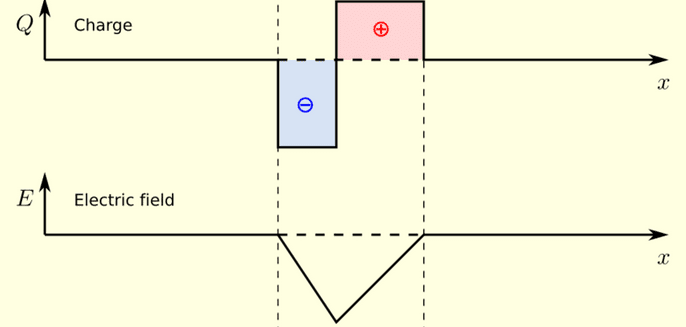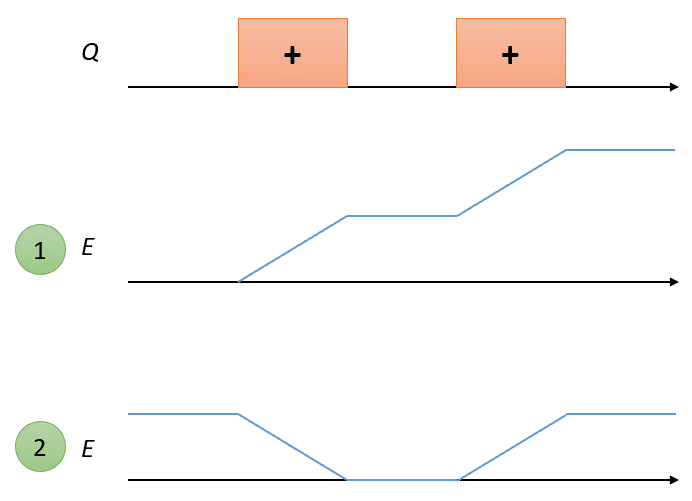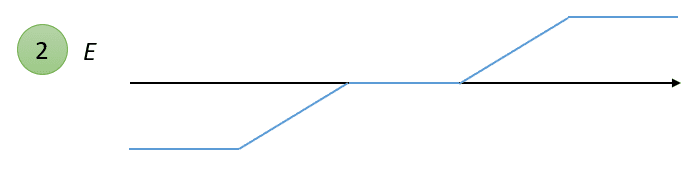# Confusion in calculating the electric field of two positive charge distributions with a gap in 1D

• I
Noki Lee
Can we apply the 1d equation (dE/dx = labmda/epsilon0)dEdx=λϵ0 to the first and the second figures?But, in the 2nd case,if we integrate the charge density, some field exists between the two charge densities. Intuitively, it should be like the last figure.
What's wrong with this?

•Delta2

Your intuition is wrong. The E-field on the left and right point in opposite directions. This is what you get in your case (1) if you add a (negative) constant of integration so E is zero between the two charges.

•Noki Lee
Noki Lee
Your intuition is wrong. The E-field on the left and right point in opposite directions. This is what you get in your case (1) if you add a (negative) constant of integration so E is zero between the two charges.I mistook the intuition, did you mean this figure?

But why we can't apply the above 1D equation?

•